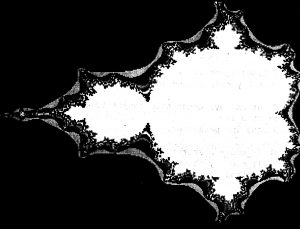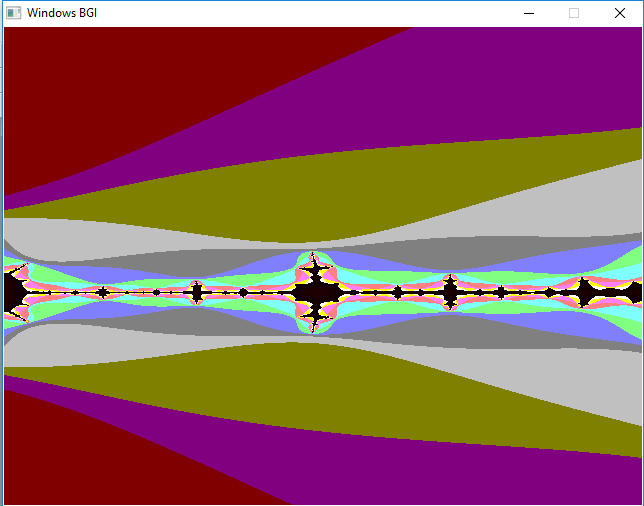Fractals in C/C++

• Difficulty Level : Medium
• Last Updated : 03 Oct, 2018

A Fractal is a never-ending pattern. Fractals are infinitely complex patterns that are self-similar across different scales. They are created by repeating a simple process over and over in an ongoing feedback loop. Mathematically fractals can be explained as follows.

• The location of a point on a screen is fed into an equation as its initial solution and the equation is iterated a large number of times.
• If that equation tends to zero (i.e. the value at the end of the iterations is smaller than the initial value), the point is coloured black.
• If the equation tends to infinity (i.e. the final value is larger than the initial value) then depending on the rate of increase (i.e. the rate at which the value tends to infinity), the pixel is painted with an appropriate colour.

Mandelbrot Set :
The Mandelbrot set is the set of complex numbers c for which the function fc(z) = z2 + c does not diverge when iterated from z = 0, i.e, for which the sequence fc(0), fc(fc(0)) etc, remains bounded in absolute value.

The Mandelbrot set is the set of values of c in the complex plane for which the orbit of 0 under iteration of the quadratic map Zn+1 = Zn2 + c remains bounded. That is, a complex number c is part of the Mandelbrot set if, when starting with Z0 = 0 and applying the iteration repeatedly, the absolute value of Zn remains bounded however large n gets. Below given is the initial image of a Mandelbrot set zoom sequence. Black points correspond to numbers that are outside the set.Properties of Mandelbrot Set :

• The Mandelbrot set is a connected set since it always have a path from one point of the set to another point of the set so that all the points in the path are also in the set.
• The Mandelbrot Set has a finite area but infinite length of border.
• The Mandelbrot set is symmetric with respect to the real axis. This means if a complex number belongs to the set then its conjugate will also belong to that set.
• The Mandelbrot set is bounded.
• The Mandelbrot set is itself similar in a non exact sense.
• The border of the Mandelbrot set is a fractal structure with not yet known fractal dimension.

Implementation : Since the concept of Fractals involves the mathematical properties of equations, the algorithm and the programs that create fractals are difficult to write and optimize. One can find many commercial software that create fractals. These programs represent some of the most optimized and probably the best fractal algorithms and implementations that have been created. Below given is the approach :

• For drawing Mandelbrot set, set the pixel that is a complex number.
• Colour the pixel if it belongs to the set.
• Iterate through every pixel and calculate the corresponding complex numbers whose result is saved in c_real for real part and c_imaginary for imaginary part.
• Calculate the Mandelbrot function which is defined as z = z*z + c where z is a complex number.
• Since complex multiplication is difficult, break the equation and calculate the sub parts i.e. the real and the imaginary part separately.
• As square of a complex number (a + ib)2 = a2 – b2 + 2abi, where a2 -b2 is the real part and 2abi is imaginary part.
• While calculating z, calculate them separately, i.e,

Z_real = z_real*z_real – z_imaginary*z_imaginary + c_real
Z_imaginary = 2*z_real*z_imaginary + c_imaginary

• Continue to calculate these values for each pixel until we reach the maximum iterations and the absolute value of z is not less than 2. Finally, we colour the pixel.
 // C++ implementation for mandelbrot set fractals#include #include #define MAXCOUNT 30  // Function to draw mandelbrot setvoid fractal(float left, float top, float xside, float yside){    float xscale, yscale, zx, zy, cx, tempx, cy;    int x, y, i, j;    int maxx, maxy, count;      // getting maximum value of x-axis of screen    maxx = getmaxx();      // getting maximum value of y-axis of screen    maxy = getmaxy();      // setting up the xscale and yscale    xscale = xside / maxx;    yscale = yside / maxy;      // calling rectangle function    // where required image will be seen    rectangle(0, 0, maxx, maxy);      // scanning every point in that rectangular area.    // Each point represents a Complex number (x + yi).    // Iterate that complex number    for (y = 1; y <= maxy - 1; y++) {        for (x = 1; x <= maxx - 1; x++)        {            // c_real            cx = x * xscale + left;              // c_imaginary            cy = y * yscale + top;              // z_real            zx = 0;              // z_imaginary            zy = 0;            count = 0;              // Calculate whether c(c_real + c_imaginary) belongs            // to the Mandelbrot set or not and draw a pixel            // at coordinates (x, y) accordingly            // If you reach the Maximum number of iterations            // and If the distance from the origin is            // greater than 2 exit the loop            while ((zx * zx + zy * zy < 4) && (count < MAXCOUNT))            {                // Calculate Mandelbrot function                // z = z*z + c where z is a complex number                  // tempx = z_real*_real - z_imaginary*z_imaginary + c_real                tempx = zx * zx - zy * zy + cx;                  // 2*z_real*z_imaginary + c_imaginary                zy = 2 * zx * zy + cy;                  // Updating z_real = tempx                zx = tempx;                  // Increment count                count = count + 1;            }              // To display the created fractal            putpixel(x, y, count);        }    }}  // Driver codeint main(){    // gm is Graphics mode which is    // a computer display mode that    // generates image using pixels.    // DETECT is a macro defined in    // "graphics.h" header file    int gd = DETECT, gm, errorcode;      float left, top, xside, yside;      // setting the left, top, xside and yside    // for the screen and image to be displayed    left = -1.75;    top = -0.25;    xside = 0.25;    yside = 0.45;    char driver[] = "";      // initgraph initializes the    // graphics system by loading a    // graphics driver from disk    initgraph(&gd, &gm, driver);      // Function calling    fractal(left, top, xside, yside);      getch();      // closegraph function closes the    // graphics mode and deallocates    // all memory allocated by    // graphics system    closegraph();      return 0;}

Output :Applications of Fractals : The basic idea of Fractals is to find regularities in the existing irregularities. Below given are some applications of Fractals :

• Fractal image compression is used in computer science, based on the facts of fractal geometry. By using this technique image is much more compressed as compared to JPEG, GIF, etc. Also, there is no pixelization when the picture is enlarged.
• To ease the study of turbulent flows, fractal representation is used. Also, fractals are used to represent porous media which is used in petroleum science.
• Fractal shaped antenna have been recently used which help in reducing the size and the weight of antennas and providing high performance.

My Personal Notes arrow_drop_up